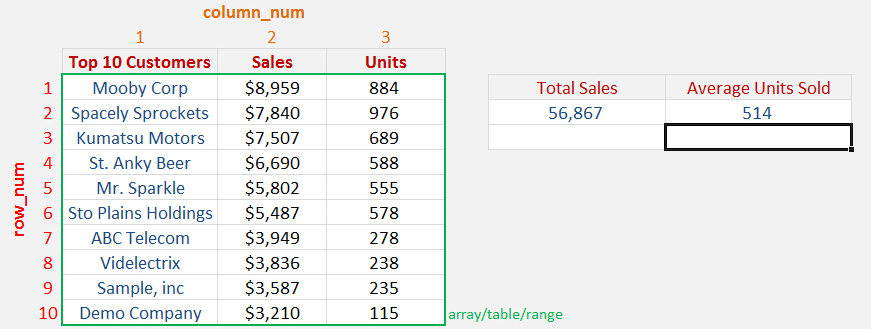You can sum a range of values within a table using the INDEX function Excel.  This is valuable when you want to extract key metrics from a table and put them in an Excel Dashboard.

To make this work you first need to start your Excel formula with the SUM Index Match.

So it will look something like this:  =SUM(INDEX(Array, Row_Num, Column_Num))

The Array will be your table of data, the Row_Num will be blank and the Column_Num will be the column number where you want to SUM the values.

When we dissect the formula (by highlighting the INDEX function and pressing F9) we can see that the following is happening: =SUM({8959;7840;7507;6690;5802;5487;3949;3836;3587;3210})So in effect, we are summing the array of values within the table.  See the example below that shows you how this is done.

### Watch Index Function Excel on YouTube and give it a thumbs-up!STEP 1: We need to place first the INDEX function inside the SUM function.

## =SUM(INDEX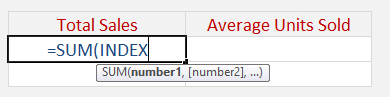The Index arguments:

## array

What is the range/table?

Reference the range of cells here that we want to get the values from:

## =SUM(INDEX(\$C\$12:\$E\$21,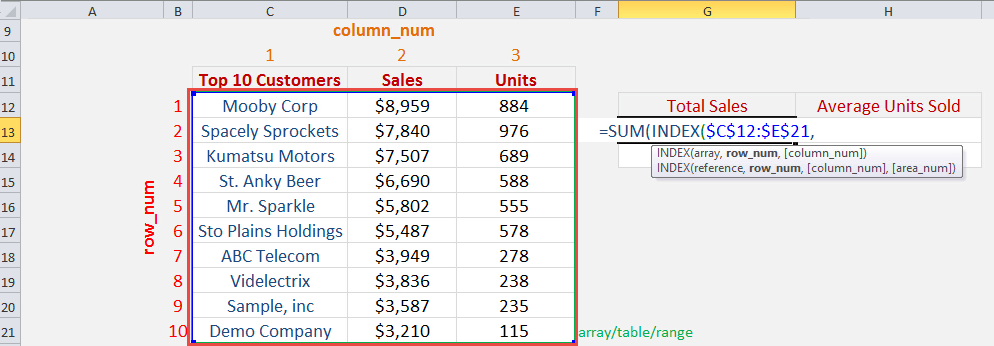## row_num

What is the row number we want to return?

We do not need to return the row, as we want to just sum all of the sales. Leave the row number blank:

## =SUM(INDEX(\$C\$12:\$E\$21,,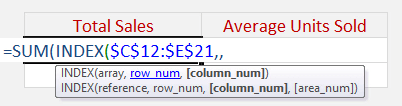## column_num

What is the column number we want to return?

Since we want the sales column, this is column number 2. So place in 2:

## =SUM(INDEX(\$C\$12:\$E\$21,,2))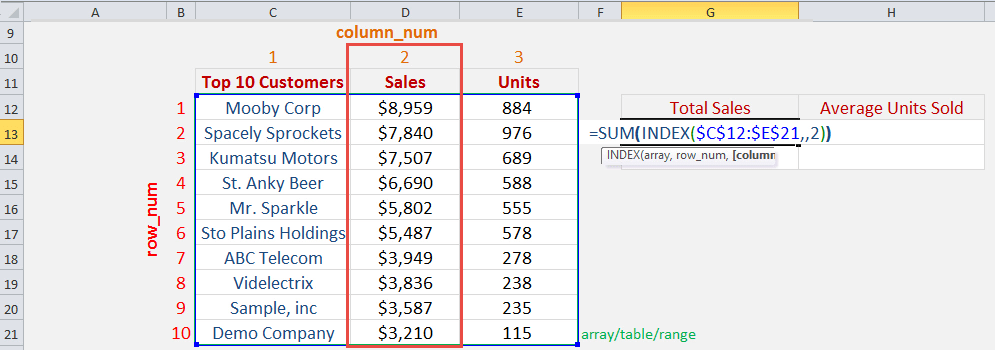Instead of providing column_num, you can also use the sum with index match function!

Now you are able to get the Total Sales: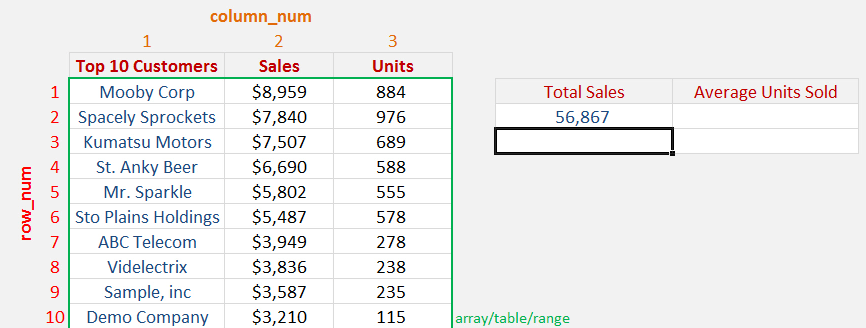STEP 2: Now let us try how we can use this with the AVERAGE function. We need to place first the INDEX function inside the AVERAGE function.

## =AVERAGE(INDEX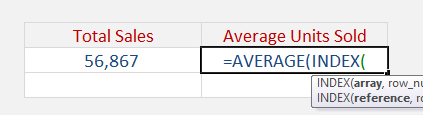The Index Function Excel arguments:

## array

What is the range/table?

Reference the range of cells here that we want to get the values from:

## =AVERAGE(INDEX(\$C\$12:\$E\$21,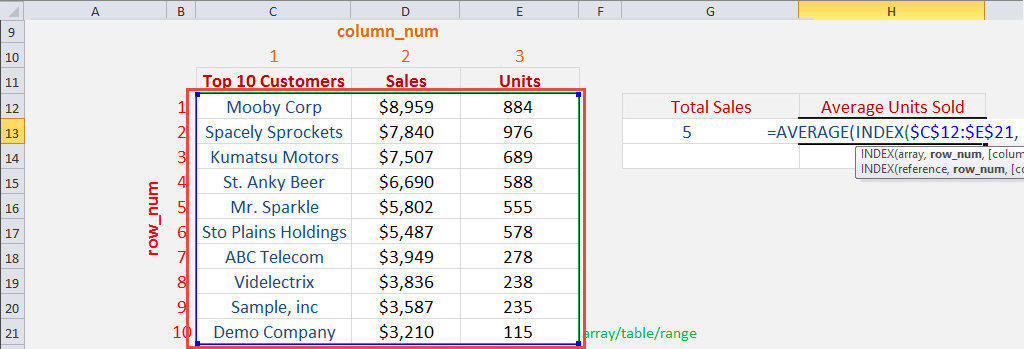## row_num

What is the row number we want to return?

We do not need to return the row, as we want to just average all of the units. Leave the row number blank:

## =AVERAGE(INDEX(\$C\$12:\$E\$21,,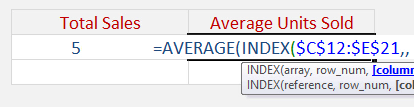## column_num

What is the column number we want to return?

Since we want the units column, this is column number 3. So place in 3:

## =AVERAGE(INDEX(\$C\$12:\$E\$21,,3))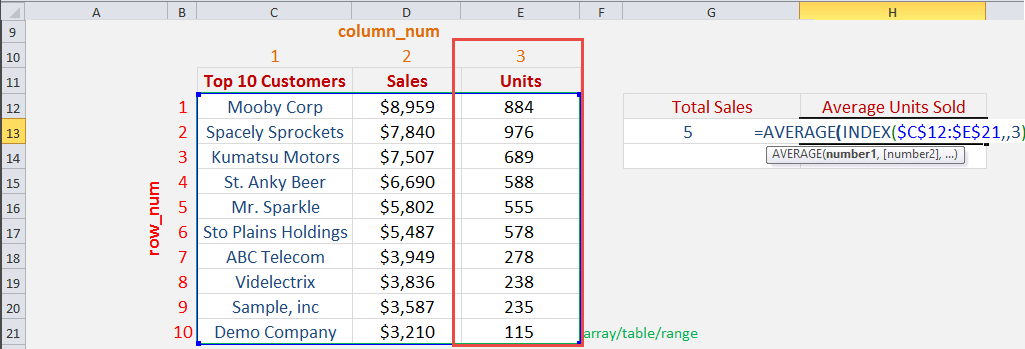Instead of providing column_num, you can also use the index match sum or index match sum multiple rows!

Now you are able to get the Average Units Sold: